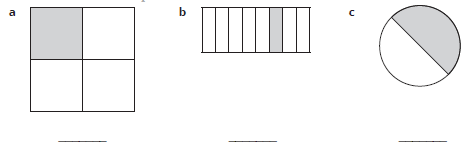# Lesson Notes By Weeks and Term - Primary 2

Fractions

TERM: 3rd Term

WEEK: 4

CLASS: Primary 2

AGE: 7 years

DURATION: 5 periods of 40 minutes each

DATE:

SUBJECT: Mathematics

TOPIC: Fractions

SPECIFIC OBJECTIVES: At the end of the lesson, the pupils should be able to

1. Identify and construct halves.
2. Identify and construct quarters.
3. Identify and construct eighths.

INSTRUCTIONAL TECHNIQUES: Explanation, question and answer, demonstration, practical

INSTRUCTIONAL MATERIALS: Paper strips (learners), large paper strips (teacher), scissors.

PERIOD 1: Assessment

PRESENTATION

TEACHER’S ACTIVITY

PUPIL’S ACTIVITY

STEP 1

ORAL

ASSESSMENTS

The teacher asks questions on mental maths treated so far

Pupils respond and participate

STEP 2

DISCUSSION

The teacher discusses all the methods used by some learners in the oral assessments(some of the questions are solved on the board by the learners) and addresses any misconceptions that may have risen

Pupils pay attention and participate

STEP 3

WRITTEN ASSESSMENTS

NOTE: Learners can draw a diagram to help them solve the word problem if they want to.

1 Complete the table.

 Answer 1. 7 × 5 = 2. 9 × 3 = 3. 9 × 4 = 4. 6 × 2 = 5. 5 × 5 = 6 8 × 2 = 7 9 × 5 = 8 7 × 4 =

2. Complete the table

 Answer is the same as 1. 5 x 3 = 15 2. 4 x 2 = 3. 5 x 4 = 4. 2 x 5 = 5. 3 x 6 =

3 There are 4 groups.

Each group has 5 children in it. How many children are there altogether? ____

Pupils attempt their class work

STEP 4

SUMMARY

The teacher marks the written assessments, corrects were necessary and commends the pupils

PERIOD 2: Fractions: Half

 PRESENTATION TEACHER’S ACTIVITY PUPIL’S ACTIVITY STEP 1MENTAL MATHS The teacher begins the lesson with some mental calculationsCalculate1 4 × 2  2 5 × 2  3 7 × 2  4 3 × 2  5 2 × 2  6 6 × 27 1 × 28 8 × 29 9 × 210 10 × 2 Pupils respond and participate STEP 2CONCEPTDEVELOPMENT The teacherAsks: Do you remember how to divide 1 loaf of bread in half? (Yes, I must cut it into 2 equal parts.)• Asks learners to draw a line through the big circle to cut the circle intotwo halves.• Gives each learner a piece of square paper to use for the following activity.• Lets the learners fold their paper in half.• Walks around and help the learners fold the paper properly (the edges of the folded paper must fit together perfectly).• Finds different ways to halve a square while you walk around and show shapes to learners.• NOTE: The importance here is to fold the page in different ways to obtain different looking halves.• Lets the learners trace the fold line with a pencil and cut the paper along the fold line.• Asks: Do you now have 2 pieces?• Lets the learners place the 2 pieces of paper on top of each other.• Asks: Are they the same size? (Yes, they are the same. Yes, they fit exactly on top of each other.)• Explains: When you make two equal parts from one thing, you call each part a (one) half of the original thing.• Writes ‘½’ and ‘half ’ on the board.• Lets the learners read ‘half ’ and write½ several times in the air.• Lets the learners write ½ in each part of the circle drawn in their classwork books.• Makes ½ of a square in the same way as learners did and paste the halves on the board (show two different shapes of halves).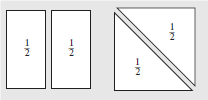• Lets the learners reconstruct the original square with their 2 halves.• Asks: What do you have now? (A square.)• Asks: Can I call the two pieces that make up the whole square shape the same name, namely one half of the square? (Yes, because when I put them together I make the square. One half of the square together with the other half of the square makes the square.)• Writes on each half of the shapes pasted on the board.• Lets the learners paste their halves into their classwork books. CLASS ACTIVITYThe teacher• Gives each learner a piece of rectangular paper and ask them to make of the rectangle.• Shows the learners how to fold it. (Show 2 different ways; along the short side and along the long side.)• Lets one learner in each of the pairs fold their paper in half along the short side and the other learner of the pair along the long side.• Lets the learners trace the fold line with a pencil and cut the paper along the fold line.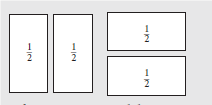• Asks: Do you now each have 2 pieces?• Lets the learners place the 2 pieces of paper on top of each other.• Asks: Are they the same size? (Yes, they are the same. Yes, they fit on top of each other.)• Asks: What do we call each of the 2 pieces? (one half)• Writes ½ on each half and paste it on the board.• Lets the learners write ½ on their halves as well.• Lets the learners read ½ several times.• Asks: Can I call these two shapes each one half of the whole rectangle? (Yes, because the two half shapes make the original rectangle when we put the two halves together.)• Lets the learners paste their halves into their classwork books. Pupils pay attention and participate STEP 3CLASS-WORK 1 Shade in ½ of each shape.2 Blessed and Tochi share 1 chocolate bar so they each get the same amount. Tochi says that they must each get one half. Is she correct? Draw a picture to show your answer. Pupils attempt their class work STEP 4HOME-WORK Draw a picture of a round cake. Share the cake equally between you and your friend Tomilola. The pupils writes it in their homework book STEP 5SUMMARY The teacher summarizes by reminding the pupils that in this lesson we have learnt to halve. She marks their class works, makes corrections where necessary and commends them positively

PERIOD 3: Fractions: Quarter

 PRESENTATION TEACHER’S ACTIVITY PUPIL’S ACTIVITY STEP 1MENTAL MATHS The teacher begins the lesson with some mental calculationsCalculate1 2 × 42 5 × 4  3 8 × 4  4 3 × 45 6 × 4  6 10 × 47 1 × 4  8 7 × 49 9 × 410 4 × 4 Pupils respond and participate STEP 2CONCEPTDEVELOPMENT The teacherUse your large strip of paper made from flip chart paper.• Folds the strip in half and then in half again.• Open the strip and trace the fold lines with a pencil.• Pastes the strip on the board.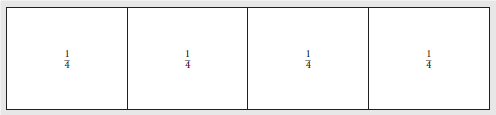• Asks: How many parts are there? (4)• Says: Let’s check whether all 4 parts are equal or not.• Cuts the strip along the lines and put the pieces on top of each other.• Says: They are all the same size. That means they are equal.• Pastes all 4 parts on the board to remake the original strip.• Explains: When you make four equal sized parts from one thing, you call each part a (one) quarter of the original thing.• Writes ¼ and ‘one quarter’ on the board.• Let the learners read ‘a quarter’ and write ¼ several times in the air.• Lets the learners write ¼ in their classwork book 4 times.• Write ¼ in each part of the strip on the board.• Explains: Numbers like ½ and ¼ are called ‘fractions’.• Lets the learners say the word ‘fractions’ several times. CLASS ACTIVITYThe teacher• Gives each learner a strip of paper that you prepared using scrap A4 paper.• Lets the learners fold the strip in half twice, as you did in Activity 1.• Lets the learners open the strip and trace the fold lines with a pencil.• Asks: How many equal parts do you see on the strip? (4)• Asks: What do we call each part of the strip? (one quarter)• Lets the learners write ¼ in each part of the strip.• Explains: We call the original strip ‘a whole’.• Lets the learners say ‘a whole’ several times.• Lets the learners paste their strips into their classwork books. Pupils pay attention and participate STEP 3CLASS-WORK Shade ¼ of each shape.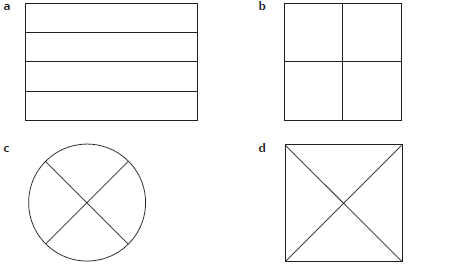Pupils attempt their class work STEP 4HOME-WORK I cut an apple into quarters. How many children can get the same size piece of the apple? The pupils writes it in their homework book STEP 5SUMMARY The teacher summarizes by reminding the pupils that in today’s lesson we have learnt what a quarter is.

PERIOD 4: Fractions-Eighth

 PRESENTATION TEACHER’S ACTIVITY PUPIL’S ACTIVITY STEP 1MENTAL MATHS The teacher begins the lesson with some mental calculationsCalculate1 8 × 22 5 × 3  3 8 × 4  4 3 × 3  5 8 × 1  6 8 × 57 7 × 48 8 × 3  9 9 × 310 5 × 4 Pupils respond and participate STEP 2CONCEPTDEVELOPMENT The teacherUse your large strip of paper made from flip chart paper.• Folds the strip in half three times.• Opens the strip and trace the fold lines with a pencil.• Pastes the strip on the board.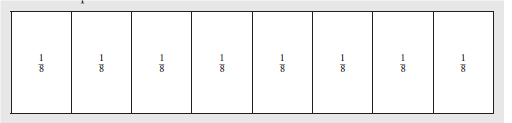• Asks: How many parts are there? (8)• Says: Let’s check whether all 8 parts are equal or not.• Cuts the strip along the lines and put the pieces on top of each other.• Says: They are all the same size. That means they are equal.• Pastes all 8 parts on the board to remake the whole strip.• Asks: What do we call the original strip? (the whole)• Explains: When you make 8 equal sized parts from a whole, you call each part an(one) eighth of the whole.• Writes ¹/8 and ‘eighth’ on the board.• Lets the learners read ‘eighth’ and write ¹/8 several times in the air.• Lets the learners write ¹/8 in their classwork book 8 times.• Write ¹/8 in each part of the strip on the board.• Asks: What do we call numbers like ½, ¼ and ¹/8 (fractions)• Lets the learners say ‘fractions’ several times. ACTIVITY IIThe teacher• Gives each learner a strip of paper that you prepared using scrap A4 paper.• Lets the learners fold the strip in half three times like you did in Activity 1.• Lets the learners open the strip and trace the fold lines with a pencil.• Asks: How many equal parts do you see on the strip? (8)• Asks: What do we call each part of the strip? (An eighth)• Lets the learners write ¹/8 in each part of the strip.• Lets the learners paste their strips into their classwork books. Pupils pay attention and participate STEP 3CLASS-WORK 1 Divide a rectangle into halves. Shade in ½2 Divide a rectangle into quarters. Shade in ¼3 Divide a rectangle into eighths. Shade in ¹/8 Pupils attempt their class work STEP 4HOME-WORK Shade in ¹/8 of each square.The pupils writes it in their homework book STEP 5SUMMARY The teacher summarizes by reminding the pupils that in today’s lesson we have learnt to identify and fold eighths.

PERIOD 5: Weekly Test/consolidations

TEACHER’S ACTIVITY: The teacher revises all the concepts treated from period 1-4 and gives the pupils follow through exercises, quiz and tests. She marks the exercises, makes corrections and commends the pupils positively.

PUPIL’S ACTIVITY: The pupils work on the worksheets and exercises given by the teacher individually

CONSOLIDATION

1 Shade in ½ of the circle.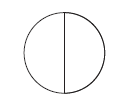2 Shade in ¼ of the circle.3 Shade in ¹/8 of the circle4 What fraction of the shape is shaded?